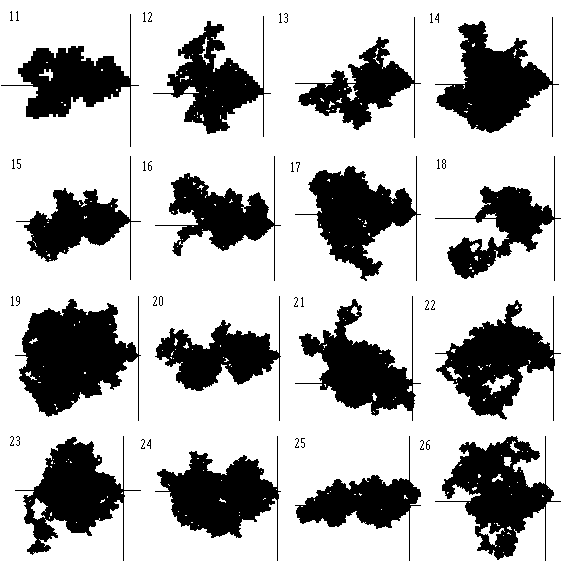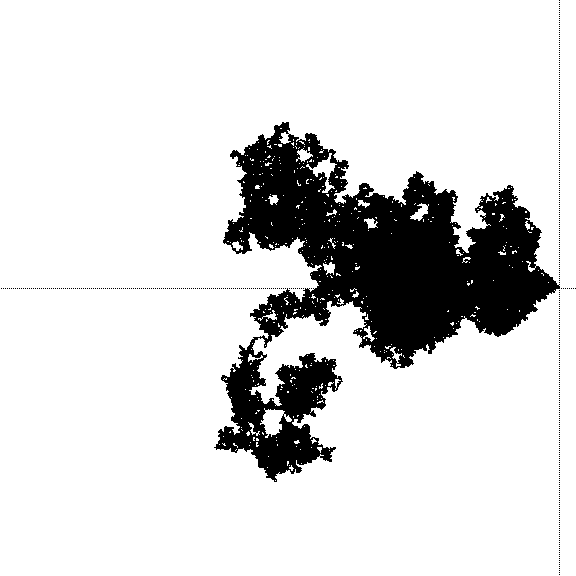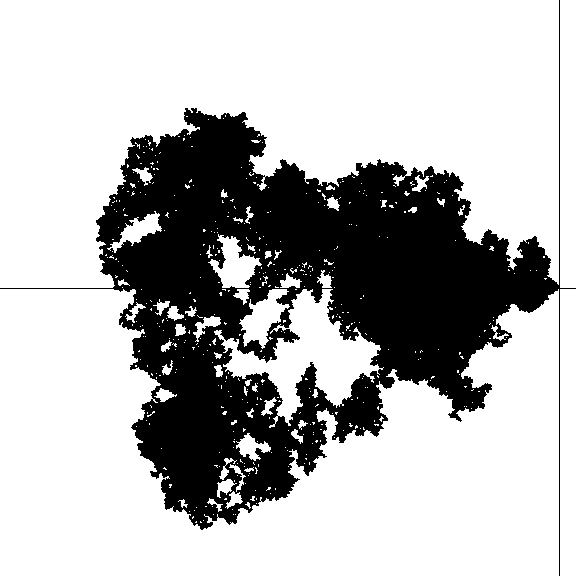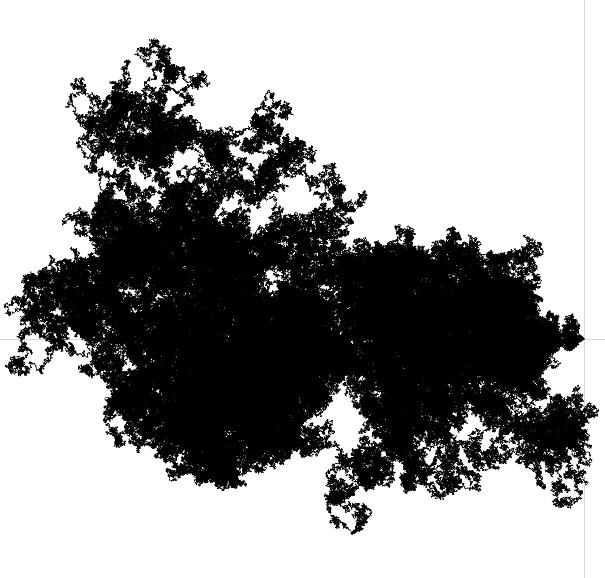# Prime number races in 2 dimensions

Is the mapping $$f: \ \mathbb{N} \rightarrow \mathbb{Z}[i], \ \ \ n \ \mapsto \sum_{2 < p \leq n \ {\rm prime}} e^{\frac{p-1}{4} \pi i}$$ surjective?

In 1999, when I was an undergraduate student, I thought about writing the thesis for my first degree on this problem. I asked Jörg Brüdern about this, and what he said was essentially that I could do this and could probably obtain some nice partial results, but that an answer would most likely be out of reach. I decided then rather to specialize in group theory.

Is it nowadays possible to say more on this question?

Plots of the images of the intervals $\{1, \dots, \lfloor e^k \rfloor\}$ for $k \in \{11, \dots, 26\}$ scaled to the same size look as follows:Larger plots of the images of the intervals $\{1, \dots, 10^k\}$ for $k \in \{7,8,9\}$ are shown below:

$k = 7$:$k = 8$:$k = 9$:• The obvious heuristic, which views this as approximately a random walk, says yes. – Will Sawin Feb 1 '13 at 22:43
• In fact, there is numerical evidence that the points in this walk 'stay much closer together' than in a random walk. Thus one might be bold to conjecture that even a 3-dimensional version of the prime race is surjective, even though the simple random walk in 3 dimensions is not recurrent. But heuristics are the one thing, rigorous proofs are the other ... . – Stefan Kohl Feb 1 '13 at 22:53
• Stefan, can you give more details about this numerical evidence? My understanding to date is that the prime number race walk is extremely well modeled by a typical random walk. – Greg Martin Feb 1 '13 at 23:19
• @Teo B: The movement in the negative and positive direction comes from the prime number race modulo $8$ between the residues $1$ and $5$. More often than not, there will be more primes congruent to $5$ modulo $8$ than $1$. (To be specific, we need to talk about the logarithmic density and assume GRH and LI) The first time that the primes congruent to $1$ modulo $8$ pulls ahead in the race is between $10^8$ and $10^9$. – Eric Naslund Feb 1 '13 at 23:38
• It's hard to give concrete reasons why the simple $\mathbb Z^2$ random walk is a suitable model for this prime race walk. (One can point to the generalized Riemann hypothesis as implying that the typical distance from the origin is on the order of the square root of the number of steps, for example.) But I feel confident in being able to deflect any specific concerns that they should be different, as I and Eric have done a little of already. Feel free to nominate other such concerns! – Greg Martin Feb 5 '13 at 1:41

This is not an answer, but rather an explanation of why this question is so difficult.

For positive coprime integers $a,q$, let $$\pi(x;q,a) = \# \{p \leq x : p \equiv a \pmod{q}\}.$$ For $k \in \mathbb{Z}$, let $$A_k = \{n \in \mathbb{N} : \pi(n;8,1) - \pi(n;8,5) = k\},$$ and let $$B_k = \{\pi(n;8,3) - \pi(n;8,7) \in \mathbb{Z} : n \in A_k\}.$$ Then your conjecture that the function $$f(n) = \sum_{p \leq n}{e^{\pi i(p - 1)/4}}$$ is surjective on $\mathbb{Z}[i]$ is equivalent to the conjecture that $B_k = \mathbb{Z}$ for each $k \in \mathbb{Z}$.

For this to happen, the set $A_k$ must be countably infinite; that is, the equality $\pi(n;8,1) = \pi(n;8,5)$ must occur infinitely often. This is a difficult result, but it is in fact known unconditionally: it is covered by Theorem 5.1 of "Comparative prime-number theory. II" by S. Knapowski, and P. Turán. Apparently, it has now been proven unconditionally by Jason Sneed that $\pi(x;q,a) - \pi(x;q,b)$ changes sign infinitely often for all $q \leq 100$, but this is yet to appear in print (see this paper for a discussion).

If one assumes two strong conjectures, the Grand Riemann hypothesis, and the Linear Independence hypothesis (namely that the imaginary parts of the nontrivial zeroes of all Dirichlet $L$-functions are linearly independent over the rationals), then one can say a lot more. Rubinstein and Sarnak's paper on Chebyshev's bias shows that not only are there infinitely many sign changes, but the function $$\left(\frac{\log x}{\sqrt{x}} \left(\pi(x;q,a_1) - \mathrm{Li}(x)\right), \ldots, \frac{\log x}{\sqrt{x}} \left(\pi(x;q,a_r) - \mathrm{Li}(x)\right)\right)$$ has a limiting logarithmic distribution. In particular, they can say roughly how likely $(\log x / \sqrt{x}) \pi(x;8,1)$ and $(\log x / \sqrt{x}) \pi(x;8,5)$ are to be in particular regions; unfortunately, this doesn't really tell you anything about the set $A_k$ for each integer $k$.

Once you have that $A_k$ is countably infinite, you still need to ensure that there is no "conspiracy" happening, in that the other prime number race $\pi(x;8,3) - \pi(x;8,7)$ could avoid certain configurations whenever $x$ is a zero of the prime number race $\pi(x;8,1) - \pi(x;8,5)$. This seems extremely difficult, and I don't know how one might attempt to analyse this. That being said, questions peripherally related to this were studied by Knapowski and Turán, so it is possible that there might be something in the literature that can deal with this type of problem.

As an aside, one interesting modification of this conjecture is the following. Let $\chi$ be a Dirichlet character modulo $q$, so that $\chi$ is generated by some root of unity $\zeta_Q$. Is the function $$f_{\chi}(n) = \sum_{p \leq n}{\chi(p)}$$ surjective on $\mathbb{Z}[\zeta_Q]$?

• The specific case of sign changes for $\pi(x;8,1) - \pi(x;8,5)$ might be among the cases proved unconditionally back before 1950 or so. – Greg Martin Feb 4 '13 at 20:37
• Regarding your $f_\chi(n)$ function: in general we shouldn't expect this to be surjective. $\mathbb Z[\zeta_q]$ will be a lattice of dimension $\phi(q)$, and the random walk analogy predicts that such a walk won't be surjective once $\phi(q)\ge3$. A related prediction would be that $f_\chi(n)$ takes the value $0$ only finitely often. A test (using a Dirichlet character modulo 11, so that $q=10$ and $\phi(q)=4$) only finds three hits $f_\chi(n)=0$ through the first $100000$ primes. (Comparison: 36 hits for a character modulo 7; 140 hits for a character modulo 5.) – Greg Martin Feb 4 '13 at 20:47
• @Greg: You are right about the first part; this was actually proved by Knapowski and Turán in 1962. I'll edit my answer accordingly. – Peter Humphries Feb 4 '13 at 21:34
• @Peter: Thank you very much for your discussion of the problem! So, to summarize, there are essentially two things which make the problem difficult: firstly the question of relations between $\pi(x;8,3) - \pi(x;8,7)$ and $\pi(x;8,1) - \pi(x;8,5)$, and secondly that information on exact numbers rather than just asymptotics is necessary. – Stefan Kohl Feb 4 '13 at 22:22